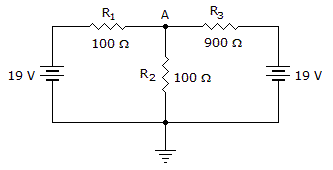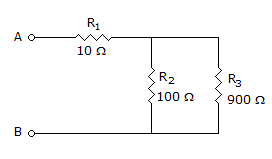# Electronics - Series-Parallel Circuits

### Exercise :: Series-Parallel Circuits - Filling the Blanks

6.In the given circuit, the voltage dropped across R1 equals _____.

 A. 1 V B. 9 V C. 10 V D. 19 V

Explanation:

No answer description available for this question. Let us discuss.

7.

___ is often used to analyze multiple-source circuits.

 A. Thevenin's theorem B. Superposition C. Kirchhoff's law D. Ohm's law

Explanation:

No answer description available for this question. Let us discuss.

8.If R3 opens in the given circuit, the total resistance (RT) between points A and B equals ____.

 A. 900B. 100C. 110D. infinite resistance

Explanation:

No answer description available for this question. Let us discuss.

9.

If a Wheatstone bridge is balanced, its output voltage is ____.

 A. the same as its input voltage B. very high C. dependent on the unknown resistor value D. equal to 0 V

Explanation:

No answer description available for this question. Let us discuss.

10.

When a load is connected to a voltage divider, the total resistance of the circuit will ________.

 A. increase B. decrease C. remain the same D. double